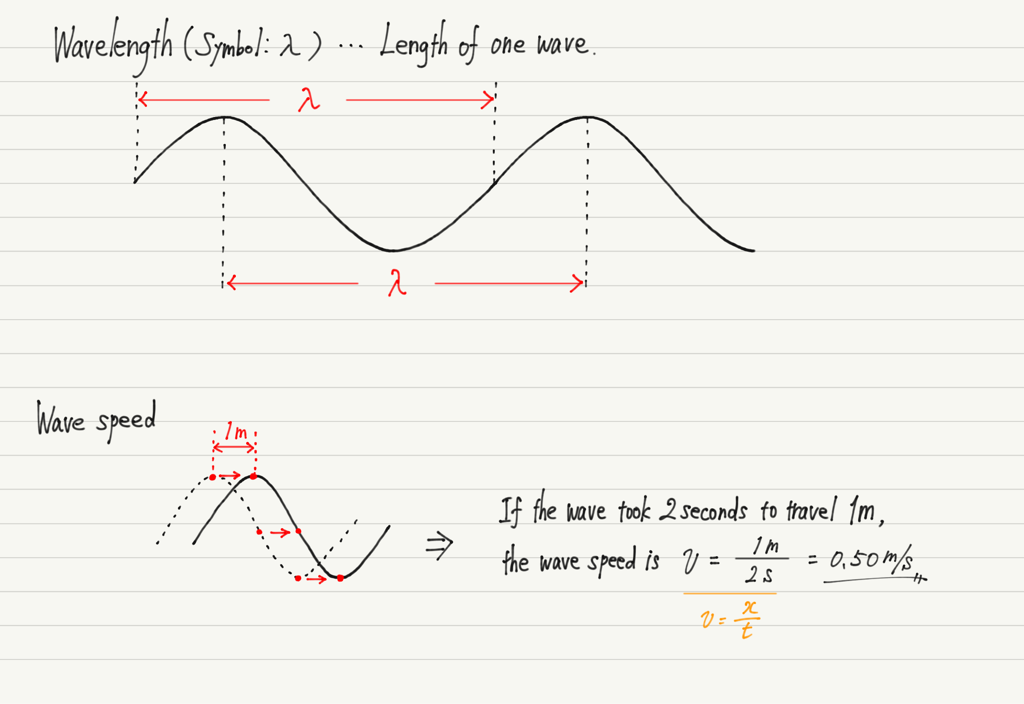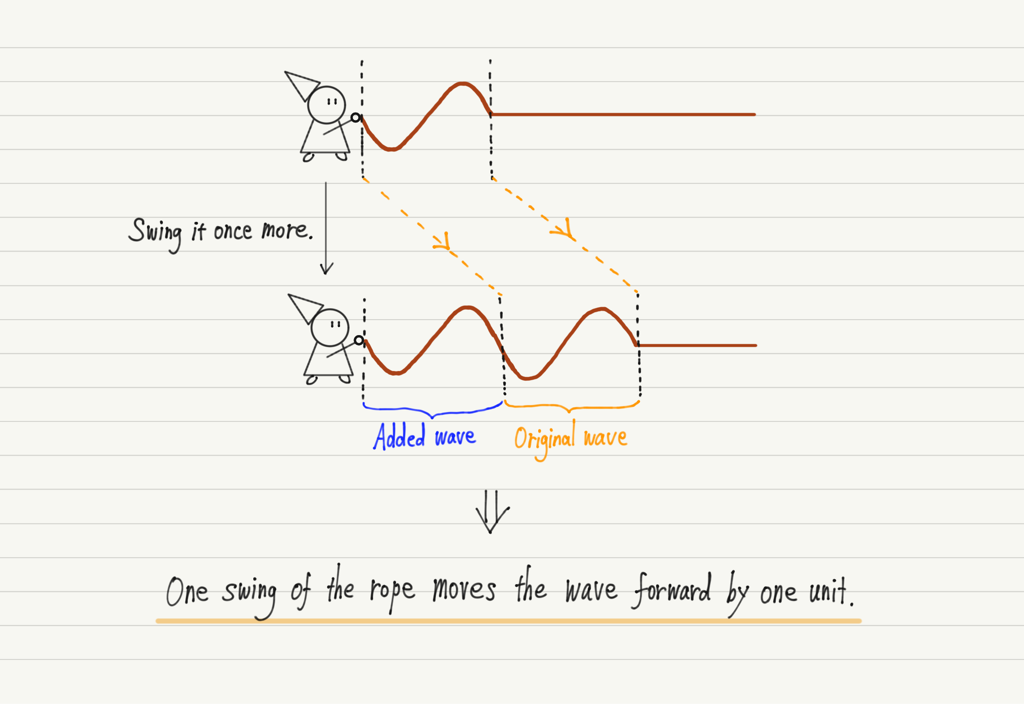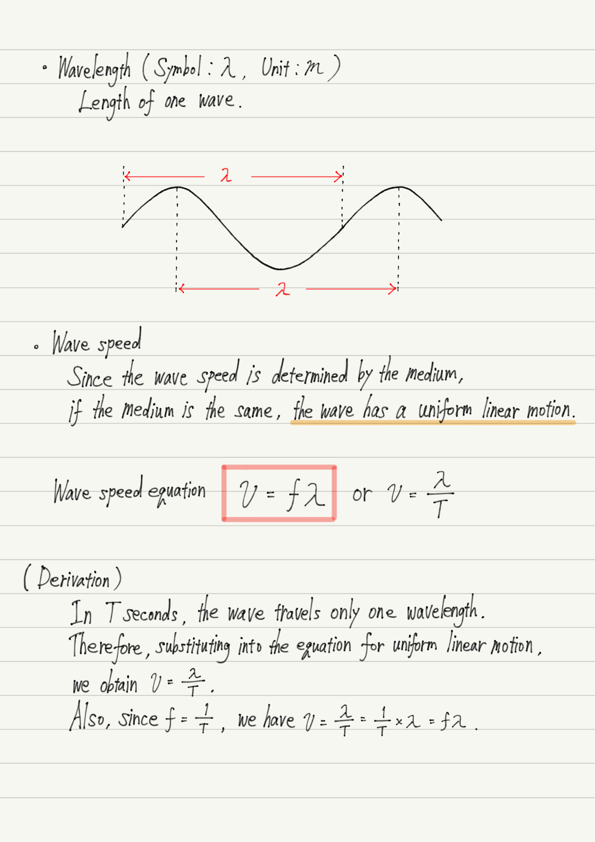# Wave Speed Equation

This time we will learn a very important equation when dealing with waves.
As in the previous lecture, we will use the example of waves propagating on a rope.

## Wavelength and Wave speed

First, a review. When the rope swings “once”, “one” wave is generated.

See previous lecture for details.

https://www.yukimura-physics.com/entry/wave-f02

The length of one wave is called wavelength.

The wave generated on the rope does not stay there, but moves toward the direction away from the hand.
The speed at which the wave moves is called the wave speed.

Since the wave speed is determined by the medium, the wave will have a uniform linear motion if it travels through the same medium.
In other words, if we know the distance traveled and the time taken, we can find the wave speed by dividing the distance by the time taken.## Wave speed equation

After swinging the rope once to create one wave, let’s continue to create another wave.
At this time, the rope will have two waves lined up.
Let us consider these two waves in line.As shown in the figure above, one swing of the rope generates one new wave.
To make room for the newly generated wave, the original wave moves forward by one wave.

Since the time required for one oscillation = period T and the length of one wave = wavelength λ, we can rephrase what we wrote above as “when time T [s] passes, the wave moves forward by λ [m]”.

And since wave speed = distance traveled / time taken, the wave speed v [m/s] can be written as v = λ / T .

Furthermore, by eliminating T using fT = 1, which we learned previous lecture, we can also write v = fλ .
This formula is very common in the field of waves, so make sure you remember it!

Incidentally, this formula, despite its importance, does not have a unique name.
Since it is inconvenient not to have a name for a formula that is used so often, this site will henceforth refer to this formula as the “Wave speed equation”!

## Summary of this lecture